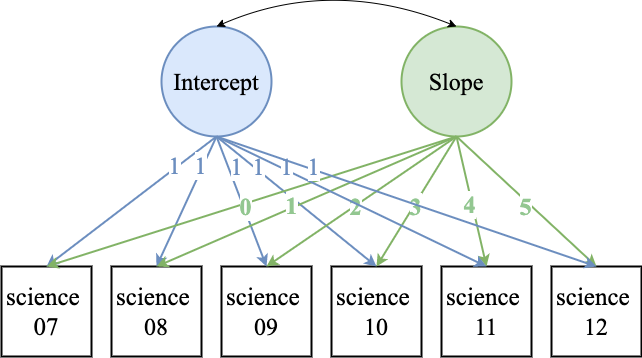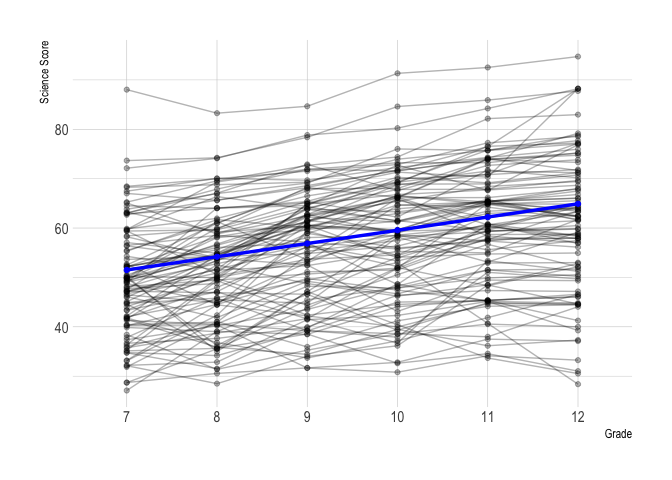# Lab 7 - Ten Growth Models - Simple to Advanced

## Structural Equation Modeling - Instructor: Karen Nylund-Gibson

### May 17, 2020

University of California, Santa Barbara

# Lab preparation

## Creating a version-controlled R-Project with Github

On the Github repository webpage:

1. fork your own branch of the lab repository
2. copy the repository web URL address from the clone or download menu

Within R-Studio:

1. click “NEW PROJECT”
2. choose option Version Control
3. choose option Git
4. paste the repository web URL path copied from the clone or download menu on Github page
5. choose location of the R-Project

## Data source:

All examples utilize the public use dataset, The Longitudinal Survey of American Youth (LSAY) $$\color{blue}{\text{See documentation here}}$$

library(transformr)
library(gganimate)
library(hrbrthemes)
library(tidyverse)
library(haven)
library(janitor)
library(MplusAutomation)
library(rhdf5)
library(here)
library(kableExtra)
library(gtsummary)
library(semPlot)
library(naniar)

## LSAY data example - Math Scores across 6 timepoints

lsay_data <- read_spss(here("data", "LSAY_labs.sav")) %>%                    #
select(RURAL, GENDER, FATHED, MOTHED,                                      #
-starts_with("AB"),                                                  #
ends_with("IMP"),                                                  #
-contains("BIO"),                                                  #
-contains("PHY")) %>%                                              #
clean_names() %>%                                                          #
rename( math_07 = amthimp ,                                                #
math_08 = cmthimp ,                                                #
math_09 = emthimp ,                                                #
math_10 = gmthimp ,                                                #
math_11 = imthimp ,                                                #
math_12 = kmthimp ,                                                #
sci_07 = asciimp ,                                                #
sci_08 = csciimp ,                                                #
sci_09 = esciimp ,                                                #
sci_10 = gsciimp ,                                                #
sci_11 = isciimp ,                                                #
sci_12 = ksciimp ) %>%                                            #
replace_with_na_all(condition = ~.x == 9999.00)                            #                    

sjPlot::view_df(lsay_data)

Write a CSV file

write_csv(lsay_data, here("data", "lsay_lab7_data.csv"))

Read in the CSV file (SPSS labels removed)

lsay_lab7 <- read_csv(here("data", "lsay_lab7_data.csv"))

# Let’s start modeling

Table. LSAY repeated measures
Name Labels Variable type
math_07 7th grade math score time varying covariate
sci_07 7th grade science score model indicators (outcomes)

## Model 01 - Fixed time effectsm1_growth  <- mplusObject(
TITLE = "m01_fixed_time_scores",
VARIABLE =
"usevar =
sci_07-sci_12; ",

ANALYSIS =
"estimator = MLR" ,

MODEL =
"i s | sci_07@0 sci_08@1 sci_09@2 sci_10@3 sci_11@4 sci_12@5; " ,

OUTPUT = "sampstat standardized;",

PLOT = "type=plot3;
series = sci_07-sci_12(*)",

usevariables = colnames(lsay_lab7),
rdata = lsay_lab7)

m1_growth_fit <- mplusModeler(m1_growth,
dataout=here("mplus_files", "Lab7.dat"),
modelout=here("mplus_files", "m1_growth_Lab7.inp"),
check=TRUE, run = TRUE, hashfilename = FALSE)

Load in the mplus.R functions

source(here("mplus.R.txt"))
##  "Loaded rhdf5 package"

Plotting using gh5 data generated by Mplus

1. View plots available for a given model
2. Generate plots using the get.plot.___ function
3. Extract data and transform to tidy format
4. Plot with ggplot
mplus.view.plots(here("mplus_files", "m1_growth_Lab7.gh5"))

Prepare plot data

observed <- lsay_lab7 %>% select(starts_with("sci")) %>%
rownames_to_column() %>% drop_na()

obs100 <- observed[1:100,]

plot_obs <- obs100 %>%
pivot_longer(sci_07:sci_12, # The columns I'm gathering together
names_to = "grade", # new column name for existing names
values_to = "value") # new column name to store values

mean_est <- as.data.frame(mplus.get.estimated_means(here(
"mplus_files", "m1_growth_Lab7.gh5"))) %>%
mutate(grade = gradelevels)

Plot the model estimated means superimposted on the obserbed individual values

growth_plot <- ggplot() +                                                                   #
geom_point(data = plot_obs,                                                               #
aes(x = grade, y = value, group = rowname), alpha = .3) +                      #
geom_line(data = plot_obs,                                                                #
aes(x = grade, y = value, group = rowname), alpha = .3) +                       #
geom_point(data=mean_est,                                                                 #
aes(x=grade, y = V1), color = "Blue", size = 1.5) +                            #
geom_line(data=mean_est,                                                                  #
aes(x=grade, y = V1, group = 1), color = "Blue", size = 1.2) +                  #
scale_x_discrete(labels = c("7", "8", "9", "10", "11", "12")) +                           #
theme_ipsum()

growth_plotggsave(here("figures", "spaghetti_p1.png"), height = 6, width = 8, dpi = "retina")

Animate the plot with {gganimate}

growth_plot +                                                                                #
transition_states(rowname,                                                                 #
transition_length = 1,                                                   #
state_length = 1) +                                                      #
shadow_mark(color = "Magenta", alpha = .3)                                                 #